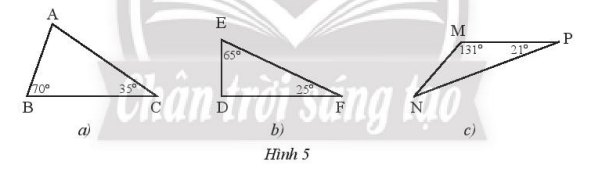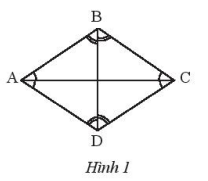## Solving SBT Lesson 1: Angles and sides of a triangle (C8 SBT Math 7 Horizons) – Math Book

Solving SBT Lesson 1: Angles and sides of a triangle (C8 SBT Math 7 Horizons)
============\

### Solution 1 page 41 SBT Math 7 Creative horizon episode 2 – CTST

Find the measure of the unknown angles of the triangles in figure 5## Detailed instructions for solving Lesson 1

Solution method

The measure of the remaining angle in a triangle is $${180^o}$$ minus the sum of the two known angles.

Detailed explanation

Considering triangle ABC, we have:

$$\widehat {{A^{}}} + \widehat B + \widehat C = {180^o}$$ so: $$\widehat {{A^{}}} = {180^o} – \widehat B – \widehat C = {180^o} – {70^o} – {35^o} = {75^o}$$

– Considering triangle EFD we have:

$$\widehat D + \widehat E + \widehat F = {180^o}$$ deduce: $$\widehat D = {180^o} – \widehat E – \widehat F = {180^o} – {65^o} – {25^o} = {90^o}$$

Considering the triangle MNP, we have:

$$\widehat M + \widehat N + \widehat P = {180^o}$$ derives: $$\widehat N = {180^o} – \widehat M – \widehat P = {180^o} – {131^o} – {21^o} = {28^o}$$

–>

— *****

### Solution 2 page 41 SBT Math 7 Creative horizon episode 2 – CTST

Find the measure of x in figure 6## Detailed instructions for solving Lesson 2

Solution method

The measure of a right angle is $${90^o}$$

The measure of the remaining angle in a triangle is $${180^o}$$ minus the sum of the two known angles

Detailed explanation

Picture A:

– Considering triangle AHC we have: $$\widehat C = {180^o} – \widehat {{A^{}}} – \widehat H = {180^o} – {61^o} – {90^ o} = {29^o}$$

Consider a triangle ABC with: $$\widehat {{A^{}}} + \widehat B + \widehat C = {180^o}$$ so: $$x = \widehat B = {180^o} – \widehat A – \widehat C = {180^o} – {90^o} – {29^o} = {61^o}$$

– Consider triangle EKG with: $$\widehat {GEK} + \widehat {EKG} + \widehat G = {180^o}$$

Derive: $$\widehat {GEK} = {180^o} – \widehat {EKG} – \widehat G = {180^o} – {90^o} – {41^o} = {49^o}$$

Which: $$\widehat {GEK} + \widehat {{\rm{KEF}}} = {90^o}$$ yields: $$x = \widehat {{\rm{KEF}}} = {90 ^o} – \widehat {GEK} = {90^o} – {49^o} = {41^o}$$

–>

— *****

### Solution 3 page 42 SBT Math 7 Creative horizon episode 2 – CTST

Find the sum of the 4 angles in a rhombus ABCD

## Detailed instructions for solving Lesson 3

Solution method

Divide the rhombus into two triangles.

Detailed explanationThe diagonal AC divides the rhombus into two triangles, we have the sum of 4 angles of a rhombus ABCD equal to the sum of the angles of two triangles ABC and ADC and equal to $${360^o}$$.

–>

— *****

### Solution 4 page 42 SBT Math 7 Creative horizon episode 2 – CTST

Which of the following triples can be the lengths of the three sides of a triangle:

a) 1 cm, 7 cm, 9 cm

b) 2 cm, 6 cm, 8 cm

c) 5 cm, 6 cm, 10 cm

## Detailed instructions for solving Lesson 4

Solution method

Check if the triple of length satisfies the triangle inequality

Detailed explanation

a) The triplet of lengths 1 cm, 7 cm, 9 cm is not the length of the three sides of a triangle because it does not satisfy the triangle inequality: 9 > 1 + 7.

b) The triplet of lengths 2 cm, 6 cm, and 8 cm is not the length of the three sides of a triangle because the triangle inequality is not satisfied: 8 = 2 + 6.

c) The triplet of lengths 5 cm, 6 cm, 10 cm can be the lengths of the three sides of a triangle because the triangle inequality is satisfied: 6 – 5 < 10 < 6 + 5

–>

— *****

### Solution 5 page 42 SBT Math 7 Creative horizon episode 2 – CTST

Let ABC be a triangle with BC = 9 cm, AB = 1 cm. Find the length of side AC, knowing this length is an integer.

## Detailed instructions for solving Lesson 5

Solution method

Using triangle inequality: BC – AB < AC < BC + AB

Detailed explanation

We have: 9 – 1 = 8 < AC < 10 = 9 +1, AC is an inferred integer AC = 9 cm

–>

— *****

### Solution 6 page 42 SBT Math 7 Creative horizon episode 2 – CTST

In a research station, people mark three areas M, N, P as the three vertices of a triangle, knowing the distances MN = 30 m, MP – 90 m.

a) If a broadcasting station is located in area P with an operating radius of 60 m, will the signal be received in area N? Why?

b) Same question as above with an operating radius of 120 m.

## Detailed instructions for solving Lesson 6

Solution method

Use the triangle inequality: MP + MN > PN > MP – MN to check the conditions.

Detailed explanation

a) Applying the triangle inequality in triangle MNP, we get:

$$\begin{array}{l}MP + MM > PN > MP – MN\\90 + 30 > PN > 90 – 30\\120 > PN > 60\end{array}$$